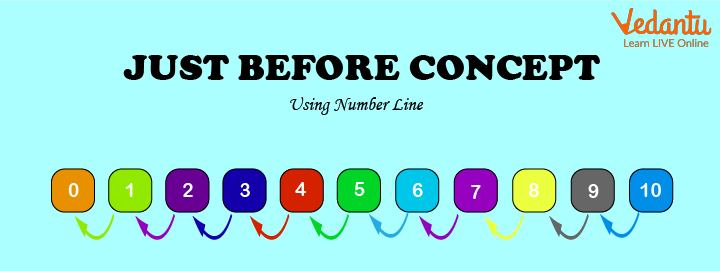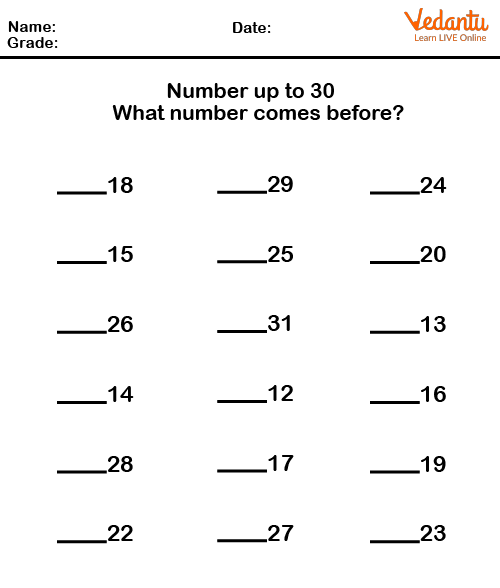Courses
Courses for Kids
Free study material
Free LIVE classes
More

# Before NumberLIVE
Join Vedantu’s FREE Mastercalss

## Introduction to Before Number

Do you know what comes before 236? We all learned counting in our previous classes. We all have learned the concept of less than, more than, and equals to. In this module, we are going to see the concept of before numbers.

Counting is a series of numbers in order. We use counting in our daily lives. We do addition, subtraction, division, and multiplication in our day-to-day life. The series of numbers have to be kept in mind for various purposes.

Every number has a number before and a number following the same. The number which comes before is the before the number and the number which follows a number is the after number. Before numbers can be easily understood through counting. A number preceding the number is before the number. For example, let's take a series of numbers which is 1,2,3,4,5,6,7,8,9. Now let's say the question is what is the previous number of 3, now if series 2 comes before 3. Hence, the before a number of 3 is 2.

## Difference between Before Number and After Number

As the before number is the number that comes before, the after number is the number that comes after the specified number. In the counting series of 1,2,3,4,5,6,7,8,9, if the before a number of 5 is 4 then the after a number of 5 is 6. Another example is the before a number of 8 is 7 and the after a number of 8 is 9.

## Before Number through Number Line Representation

Numbers can also be understood through Number Line Representation.Just Before Concept

The number which comes left to the number is before the number. Let's take an example through this number line representation. As we can clearly see the arrows which are depicting the before numbers. 9 comes before 10, 8 comes before 9, 7 comes before 8, 6 comes before 7, 5 comes before 6, 4 comes before 5, 3 comes before 4, 2 comes before 3, and 1 comes before 2. This table can also be used to understand the concept of after number. 2 comes after 1, 3 comes after 2, 6 comes after 5, etc.

## Solved Examples

Q1. What comes before 18?

Ans: Let’s memorise the counting to find the answer to this question. The answer to this would be 17 as 17 comes before 18 in the counting series. In the same way, the answer to what comes after 18 would be 19.

Q2. What comes before 15?

Ans: To solve this question, we have to keep counting in mind. As 14 comes before 15 in the counting. 14 is before a number of 15.Worksheet for Before Number

This worksheet will help you understand the concept of before numbers in a better way. Children have to solve all the given questions.

Q1. What comes before 675?

Ans: 674 comes before 675.

Q2. What comes before and after 238?

Ans: 237 comes before 238 and 239 comes after 238.

## Summary

We all know the concept of counting which is used in addition, subtraction, multiplication, and division. The concept of counting is used to identify before numbers as well. Before number is the number that comes before the specified number. Before numbers are also used in more than and less than concepts of numbers.

Last updated date: 04th Oct 2023
Total views: 125.4k
Views today: 4.25k

## FAQs on Before Number

1. Describe the concepts of before number and after the number? Give some examples as well?

We have to know counting to understand the concept of before numbers and after numbers. By the general understanding, we know that before comes first and after comes later. The same happens in counting, the number which comes earlier is before and the number which comes later is after. Let us take a simple example, of the digit 5 which comes before in the counting. By repeating the counting, 1,2,3,4,5,6, we know that 4 comes before 5. We also know the number coming later to 5 which is 6, so 6 is the after the number.

2. How is the concept of between numbers different from before numbers?

Before is what comes early in the series of counting to the specified number. A predecessor to the number is before the number. On the other hand, between numbers falls in between two numbers. To find a between-number, we require two numbers, but only a single number is enough to find the before the number. Let us take an example to understand it better. What comes before 8 and what comes between 8 and 10, to answer this question we need to know both the concepts. 7 is before a number of 8 and 9 comes between 8 and 10 as per the counting.